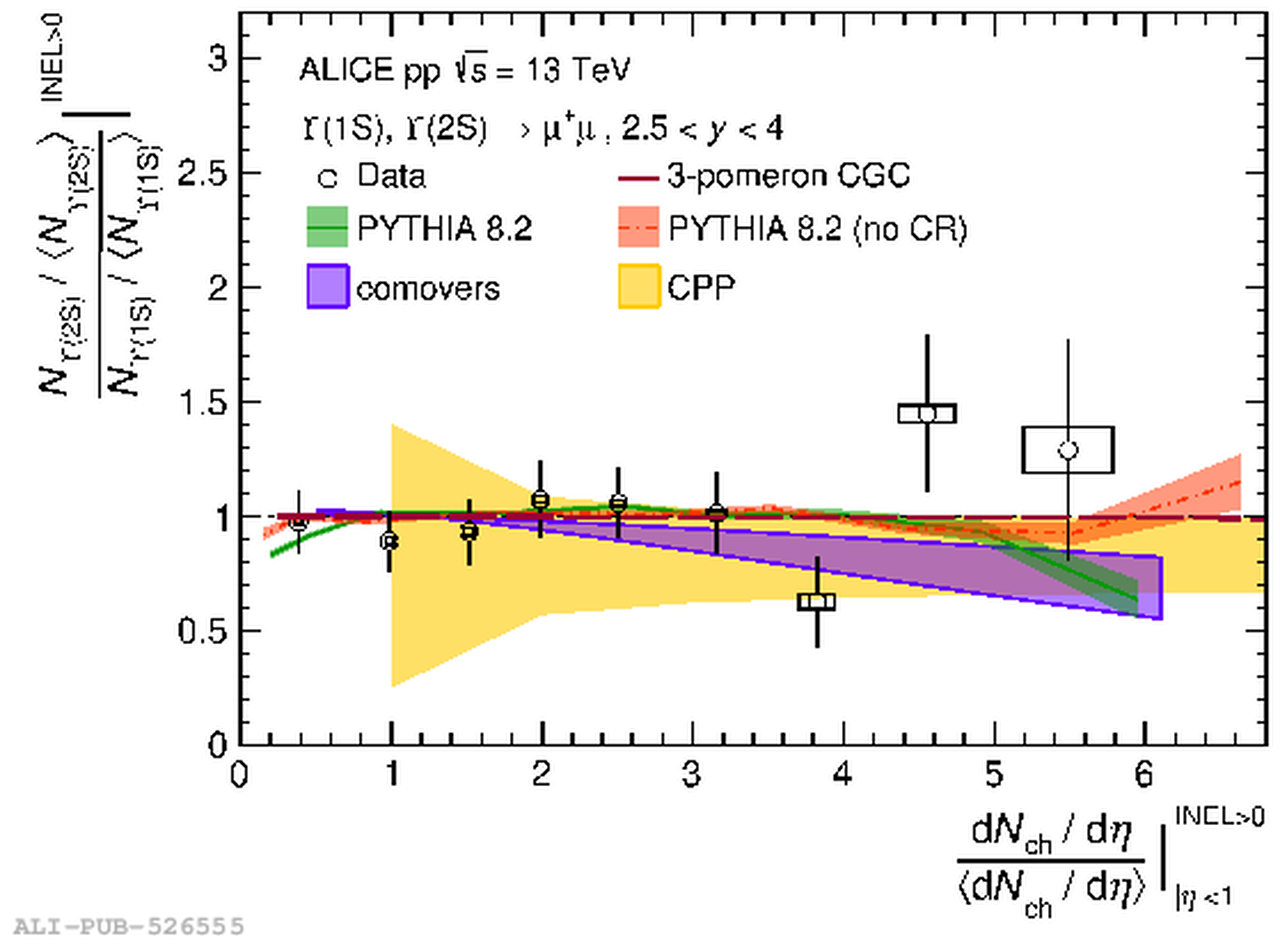# Figure 4

 Top: Excited-to-ground state self-normalized yield ratio ($\Upsilon$(2S) over $\Upsilon$(1S)) as a function of self-normalized charged-particle multiplicity, compared to model predictions from 3-pomeron CGC approach , PYTHIA 8.2 , comovers , and CPP  predictions; Bottom: Excited-to-ground state self-normalized yield ratio ($\Upsilon$(3S) over $\Upsilon$(1S)) as a function of self-normalized multiplicity, compared to PYTHIA 8.2 and comovers predictions..